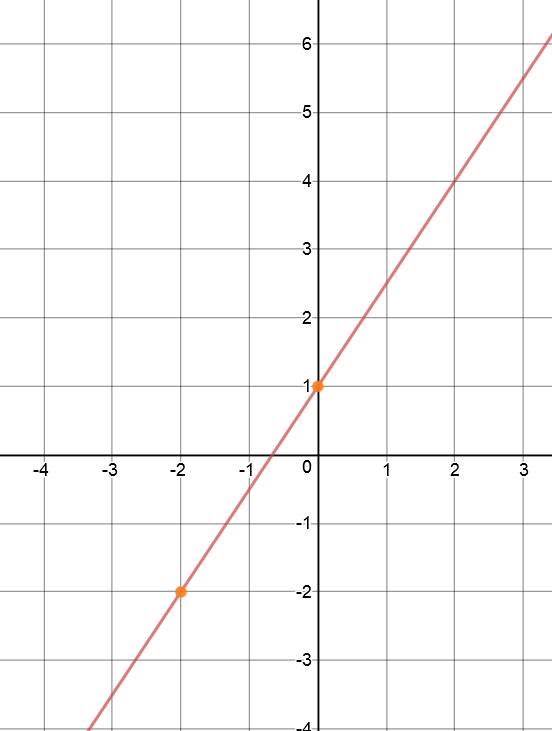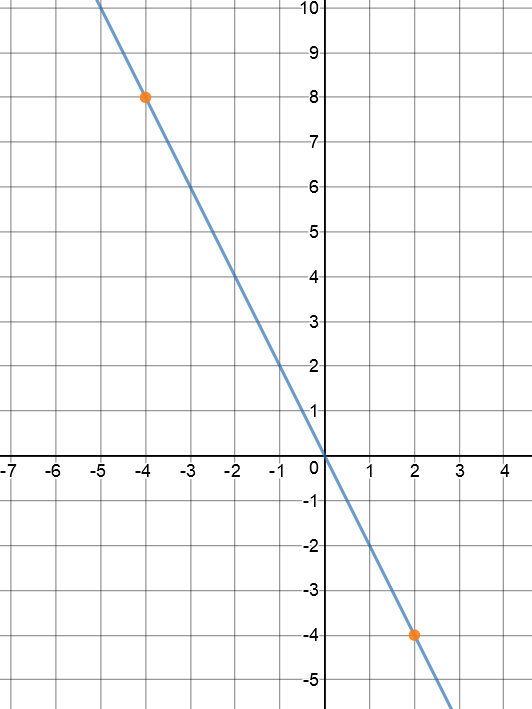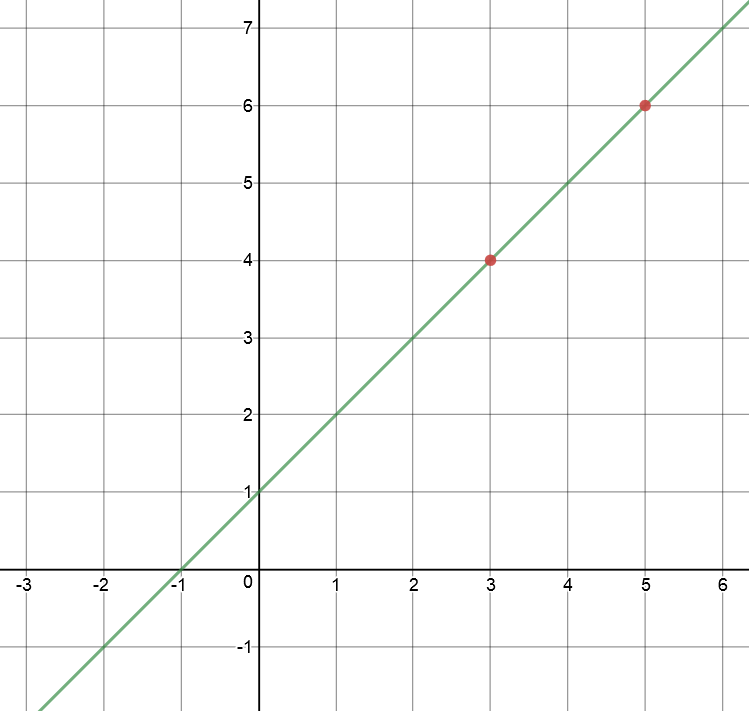# Equation of a Straight Line From Two Points

## A Little Bit of NonsenseMeet Edward the Echidna. Being a very mathematically-minded monotreme, he has decided to model the growth of the termite mounds in his neighbourhood. He has observed that the height of termite mounds grows linearly as a function of the base radius, and that harvesting them is most efficient when their base radius measures 20 snouts. A "snout" is a standard echidna unit of measurement. He wants to know what the height of a newly discovered termite mound will be when the base radius is 20 snouts. After several weeks of observation, he knows that, when the base radius measured 3 snouts, the height of the mound was 4 snouts, and when the base radius measured 4 snouts, the height of the mound was 5 snouts.

Putting this all together, we can see that Edward is looking for the equation of a straight line which passes through the points $(3,4)$ and $(4,5)$. He will then need to substitute in the value $\text{radius} = 20$ to find the height of the termite mound. But how can he find this equation? We know how to find the equation if we know the gradient and the $y$-intercept of the line, or the gradient and a point on the line. How can we find it if we only know two points on the line?

Actually, there's another version of the equation of a straight line that can help Edward out (and, being stubborn, I claim that you don't need it. More of that later!).

## Deriving the Equation of a Line from Two Points

We've already seen the point-gradient equation for a straight line. In case you've forgotten, it's:

$y - y_1 = m(x - x_1).$
But what if we only know the two points $(x_1,y_1)$ and $(x_2,y_2)$, and not the gradient of the line? Well, we can work out what the gradient is, and then we'll have all the information we need to find the equation of the line. Let's hop to it (do echidnas hop? Maybe "scurry" is a better word.):
$m = \text{gradient} = \dfrac{\text{change in y}}{\text{change in x}} = \dfrac{y_2 - y_1}{x_2 - x_1}.$
It looks like our two points give us all the information we need to find the point-gradient equation of the line:
\begin{align*} y - y_1 &= m(x - x_1)\\ y - y_1 &= \left(\dfrac{y_2 - y_1}{x_2 - x_1} \right) (x - x_1). \mbox{ Now tidy things up by dividing both sides by } (y_2 - y_1)\\ \dfrac{y - y_1}{y_2 - y_1} &= \dfrac{x - x_1}{x_2 - x_1} \end{align*}
So the equation we're after is
$\dfrac{y - y_1}{y_2 - y_1} = \dfrac{x - x_1}{x_2 - x_1}.$

I hope someone is screaming that I could have just divided by zero. They'd be absolutely correct, but if $y_2 - y_1 = 0$, then $y_2 = y_1$ and our line must be vertical. We already know that the gradient of a vertical line is undefined, and we need to come up with a more cunning plan to write down its equation. So, everything is fine. This is the equation for a line that is not vertical and passes through the two points $(x_1,y_1)$ and $(x_2,y_2)$.

Before we apply our equation to Edward's termite mound, let's have a look at a few examples.

### Example 1

Find the equation of the line through the points $(0,1)$ and $(-2, -2)$.

Solution:

Set $(x_1,y_1) = (0,1)$ and $(x_2,y_2) = (-2,-2)$. Then

\begin{align*} \dfrac{y - y_1}{y_2 - y_1} &= \dfrac{x - x_1}{x_2 - x_1}\\ \dfrac{y - 1}{-2 - 1} &= \dfrac{x - 0}{-2 - 0}\\ \dfrac{y - 1}{-3} &= \dfrac{x}{-2}\\ y - 1 &= \dfrac{3}{2}\;x\\ y &= \dfrac{3}{2}\;x + 1 \end{align*}
So the graph looks like this:### Example 2

Find the equation of the line through the points $(-4,-1)$ and $(-2, 0)$.

Solution:

Set $(x_1,y_1) = (-4,-1)$ and $(x_2,y_2) = (-2,0)$. Then

\begin{align*} \dfrac{y - y_1}{y_2 - y_1} &= \dfrac{x - x_1}{x_2 - x_1}\\ \dfrac{y - 0}{-1 - 0} &= \dfrac{x - (-2)}{-4 - (-2)}\\ \dfrac{y}{-1} &= \dfrac{x + 2}{-2}\\ 2y &= x + 2\\ y &= \dfrac{1}{2}\;x + 2 \end{align*}
So the graph looks like this:### Example 3

Find the equation of the line through the points $(2,-4)$ and $(-4, 8)$.

Solution:

Set $(x_1,y_1) = (2,-4)$ and $(x_2,y_2) = (-4,8)$. Then

\begin{align*} \dfrac{y - y_1}{y_2 - y_1} &= \dfrac{x - x_1}{x_2 - x_1}\\ \dfrac{y - (-4)}{8 - (-4)} &= \dfrac{x - 2}{-4 -2}\\ \dfrac{y + 4}{12} &= \dfrac{x - 2}{-6}\\ y + 4 &= -2x + 4\\ y &= -2x \end{align*}
So the graph looks like this:### Now It's Time to Solve Edward's Problem

Do you remember that Edward wanted to find the height of his termite hill when its base radius measured 20 snouts? He knew that the height was 4 snouts when the base radius was 3 snouts and the height was 6 snouts when the base radius was 5 snouts. The height grew linearly with respect to the base radius, so he was looking for the equation of a straight line through the points $(3,4)$ and $(5,6)$. Using our new formula, and setting $(\text{radius}_1,\text{height}_1) = (3,4)$ and $(\text{radius}_2,\text{height}_2) = (5,6)$, we obtain

\begin{align*} \dfrac{\text{height} - \text{height}_1}{\text{height}_2 - \text{height}_1} &= \dfrac{\text{radius} - \text{radius}_1}{\text{radius}_2 - \text{radius}_1}\\ \dfrac{\text{height} - 4}{6 - 4} &= \dfrac{\text{radius} - 3}{5 -3}\\ \dfrac{\text{height} - 4}{2} &= \dfrac{\text{radius} - 3}{2}\\ \text{height} - 4 &= \text{radius} - 3\\ \text{height} &= \text{radius} + 1 \end{align*}
So the graph looks like this:and to find the height of the termite mound when the base radius is 20 snouts, we plug in $\text{radius} = 20$ to give
$\text{height} = 20 + 1 = 21 \text{ snouts}.$
So, Edward's termite mound will be $21$ snouts high.

## The Alternative Approach

I'm allergic to memorising too many formulas, and I think that it's enough to memorise $y = mx + b$ as the equation of a straight line.

Here's what I do:

1. Plug the two points into the equation $y = mx + b$ individually, to give two equations in $m$ and $b$.
2. Solve the two equations simultaneously to find $m$ and $b$.
3. Write down the equation of the line in the form $y = mx + b$.

Let's look at an example. It's just Edward's problem rephrased.

### Example 5

Find the equation of the line through the points $(3,4)$ and $(5,6)$.

Solution:

1. Plug the two points into the equation $y = mx + b$ individually, to give two equations in $m$ and $b$.
• $(3,4)$ : $y = 4$ and $x = 3$, so $4 = 3m + b$
• $(5,6)$ : $y = 6$ and $x = 5$, $6 = 5m + b$
2. Solve the two equations simultaneously to find $m$ and $b$.
Subtracting the second equation from the first yields
\begin{align*} 6 - 4 &= (5 - 3)m + (b - b)\\ 2 &= 2m\\ 1 &= m \end{align*}
Now plug $m = 1$ into the first equation to find $b$:
\begin{align*} 4 &= 3(1) + b\\ 4&= 3 + b\\ 1 &= b \end{align*}
3. Write down the equation of the line in the form $y = mx + b$.
\begin{align*} y &= mx + b\\ y &= x + 1 \end{align*} So, the equation is $y = x + 1$, which is exactly what we got using the other approach.

I'll leave it to you to choose the approach you wish to follow. In another article we'll show how to find the equation of a line through two points using the point-gradient formula.

### Description

coordinate geometry is a branch of geometry where the position of the points on the plane is defined with the help of an ordered pair of numbers also known as coordinates. In this tutorial series, you will learn about vast range of topics such as Cartesian Coordinates, Midpoint of a Line Segment etc

### Audience

year 10 or higher, several chapters suitable for Year 8+ students.

### Learning Objectives

Explore topics related to Coordinates Geometry

Author: Subject Coach
You must be logged in as Student to ask a Question.